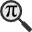## General

Display information for equation id:math.242012.2 on revision:242012

* Page found: Radial basis function (eq math.242012.2)

(force rerendering)

Cannot find the equation data in the database. Fetching from revision text.

Occurrences on the following pages:

Hash: 1ed346930917426bc46d41e22cc525ec

TeX (original user input):

\phi


TeX (checked):

\phi


### LaTeXML (experimental; uses MathML) rendering

MathML (476 B / 243 B) :${\displaystyle\phi}$
<math xmlns="http://www.w3.org/1998/Math/MathML" id="p1.1.m1.1" class="ltx_Math" alttext="{\displaystyle\phi}" display="inline">
<semantics id="p1.1.m1.1a">
<mi id="p1.1.m1.1.1" xref="p1.1.m1.1.1.cmml">ϕ</mi>
<annotation-xml encoding="MathML-Content" id="p1.1.m1.1b">
<ci id="p1.1.m1.1.1.cmml" xref="p1.1.m1.1.1">ϕ</ci>
</annotation-xml>
<annotation encoding="application/x-tex" id="p1.1.m1.1c">{\displaystyle\phi}</annotation>
</semantics>
[/itex]


SVG (1.278 KB / 782 B) :

### MathML with SVG or PNG fallback (recommended for modern browsers and accessibility tools) rendering

SVG image empty. Force Re-Rendering

SVG (0 B / 8 B) :

PNG (0 B / 8 B) :

$\phi$## Translations to Computer Algebra Systems

### Translation to Maple

In Maple: phi

\phi: Could be the golden ratio == golden mean == golden section == extreme and mean ratio == medial section == divine proportion == divine section == golden proportion == golden cut == golden number.

But this system don't know how to translate it as a constant. It was translated as a general letter.


### Translation to Mathematica

In Mathematica: \[Phi]

\phi: Could be the golden ratio == golden mean == golden section == extreme and mean ratio == medial section == divine proportion == divine section == golden proportion == golden cut == golden number.

But this system don't know how to translate it as a constant. It was translated as a general letter.


## Similar pages

Calculated based on the variables occurring on the entire Radial basis function page

## Identifiers

• $\phi$### MathML observations

0results

0results

no statistics present please run the maintenance script ExtractFeatures.php

0 results

0 results